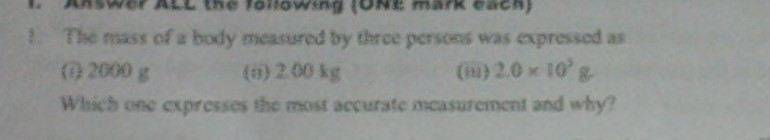Significant figures

Gold Member

Homework Statement

If they ask me to find which value is more accurate:
a)2000g
b)2.00Kg?

The Attempt at a Solution

It should be b right? The book says a,
2000g = 2Kg or is it
2000g = 2.000Kg?

Quantum Defect
Homework Helper
Gold Member

Homework Statement

If they ask me to find which value is more accurate:
a)2000g
b)2.00Kg?

The Attempt at a Solution

It should be b right? The book says a,
2000g = 2Kg or is it
2000g = 2.000Kg?

Your choice of the word "accurate" is problematic, but I think I know what is going on.

If the actual question is written as in (3), the correct answer would be 2 kg. Trailing zeros are not significant, if there is no decimal point. If the number had been written as 2000. g, then the correct answer would be 2.000 kg, but as the number was written as 2000 g, the trailing zeros are not significant.

Gold Member
Your choice of the word "accurate" is problematic, but I think I know what is going on.not my words! :)

NascentOxygen
Staff Emeritus
I'll leave it for someone else to answer definitively.

But suppose the first one was 2001 g. You would then say it was the more accurate (or more precise).

Quantum Defect
Homework Helper
Gold Member
View attachment 79547 not my words! :)

This looks really bad. "Accuracy" refers to how close to the truth the actual measurement is. If the actual mass of the object is 50 kg, all of these measurements are bad. If they are asking which measurment was made with more precision, then I would say (b), since this has the largest number of sig figs.

This just looks like a very bad question!

Gold Member
I understand, it is a bad question.
About precision, my physics book says that precision is more significant figure vut my chemistry book, it says its the closeness of many observations. Is that right too?

BvU
Homework Helper
The exercise author's choice of the value is very unfortunate. Weighing a 2 kilogram calibrated weight on an electronic (or mechanic) scale gives results like 1993, 2001, 2003 or something. With a 1% accurate scale (pretty good), chances to get exactly 2000. are really small.

If someone reports the result as 2000 g we in fact don't know if the thousand is a number or a scale factor (*). You'd have to know if it's a salesperson (scale factor) reporting that or an engineer (number, hopefully, but even engineers aren't that precise in their language).

In physics and sometimes in adjacent sciences we have a loose and silent convention that if no error is given, the last digit provided is significant and correct.
So 101.4 means 'in the range 101.35 -- 101.45". But, as QD says, the trailing zeroes are often insignificant, even in science parlance.

Compare the big cities here. No way any of these cities has e.g. between 9 999 999.5 and 10 000 000.5 inhabitants on January 1, 2015 at 0:00:00.00 hr (midnight) !

Conclusion: the book has missed an opportunity to let students actually learn this in the right way. Good students run into trouble, bad students don't hesitate and get rewarded when they pick (i).

Suraj is in the first category and senses where the snag is. At the (*)